NCERT Solutions for Class 8 Maths Chapter 14 Factorisation Ex 14.1 are part of NCERT Solutions for Class 8 Maths. Here we have given NCERT Solutions for Class 8 Maths Chapter 14 Factorisation Ex 14.1.

 Board CBSE Textbook NCERT Class Class 8 Subject Maths Chapter Chapter 14 Chapter Name Factorisation Exercise Ex 14.3 Number of Questions Solved 5 Category NCERT Solutions

## NCERT Solutions for Class 8 Maths Chapter 14 Factorisation Ex 14.3

Question 1.
Carry out the following divisions: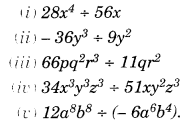Solution.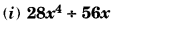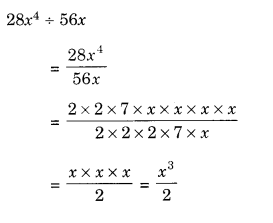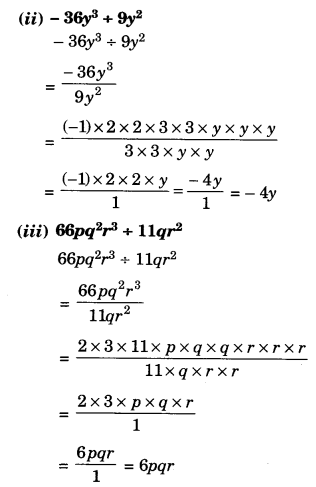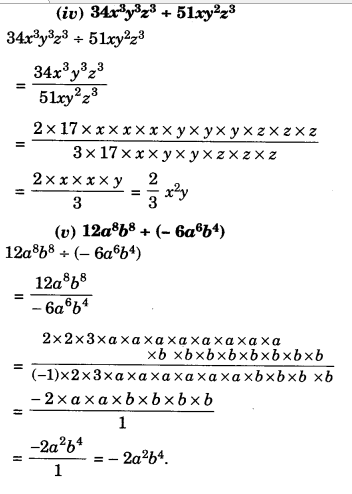Question 2.
Divide the given polynomial by the given monomial: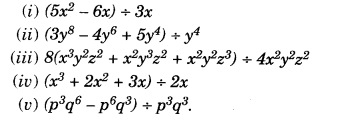Solution.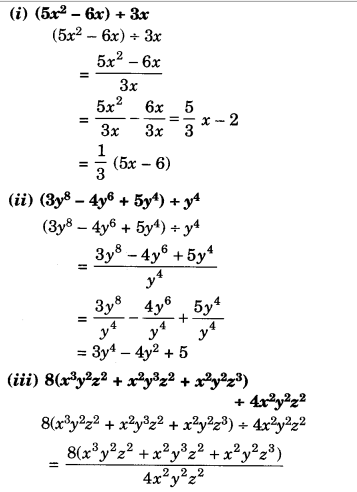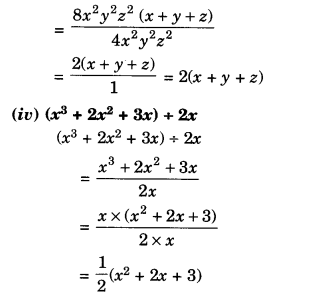Question 3.
Work out the following divisions:Solution.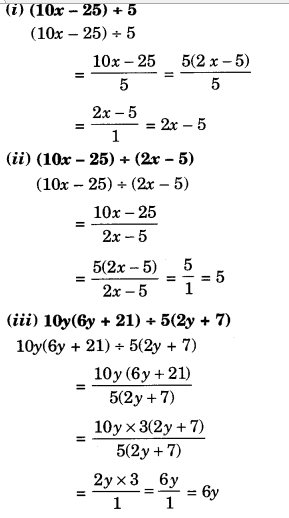Question 4.
Divide as directed.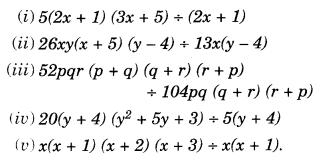Solution.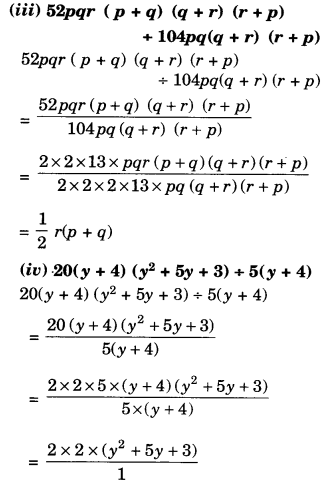Question 5.
Factorise the expressions and divide them as directed.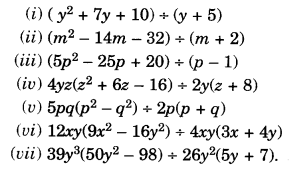Solution.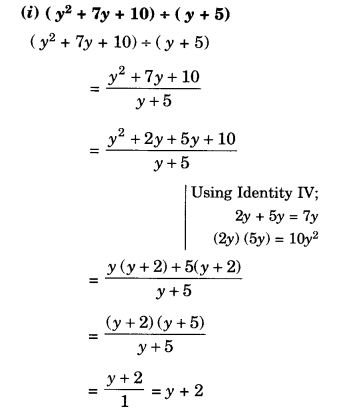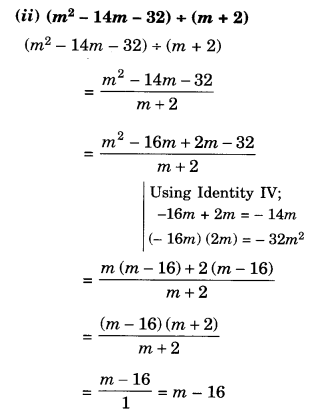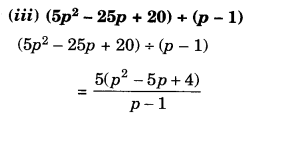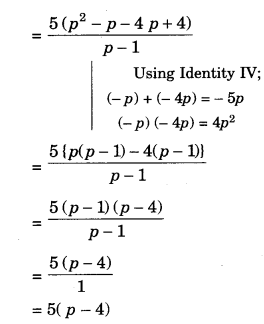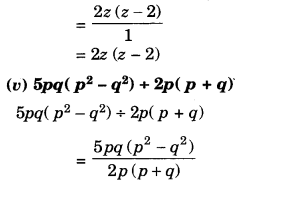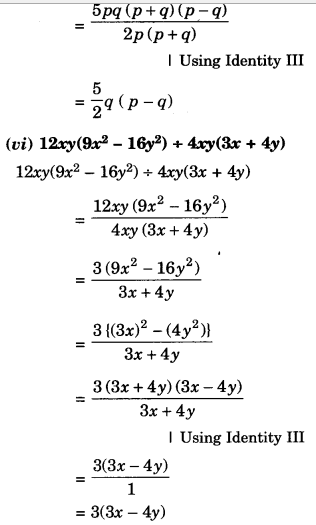We hope the NCERT Solutions for Class 8 Maths Chapter 14 Factorisation Ex 14.3 help you. If you have any query regarding NCERT Solutions for Class 8 Maths Chapter 14 Factorisation Ex 14.3, drop a comment below and we will get back to you at the earliest.